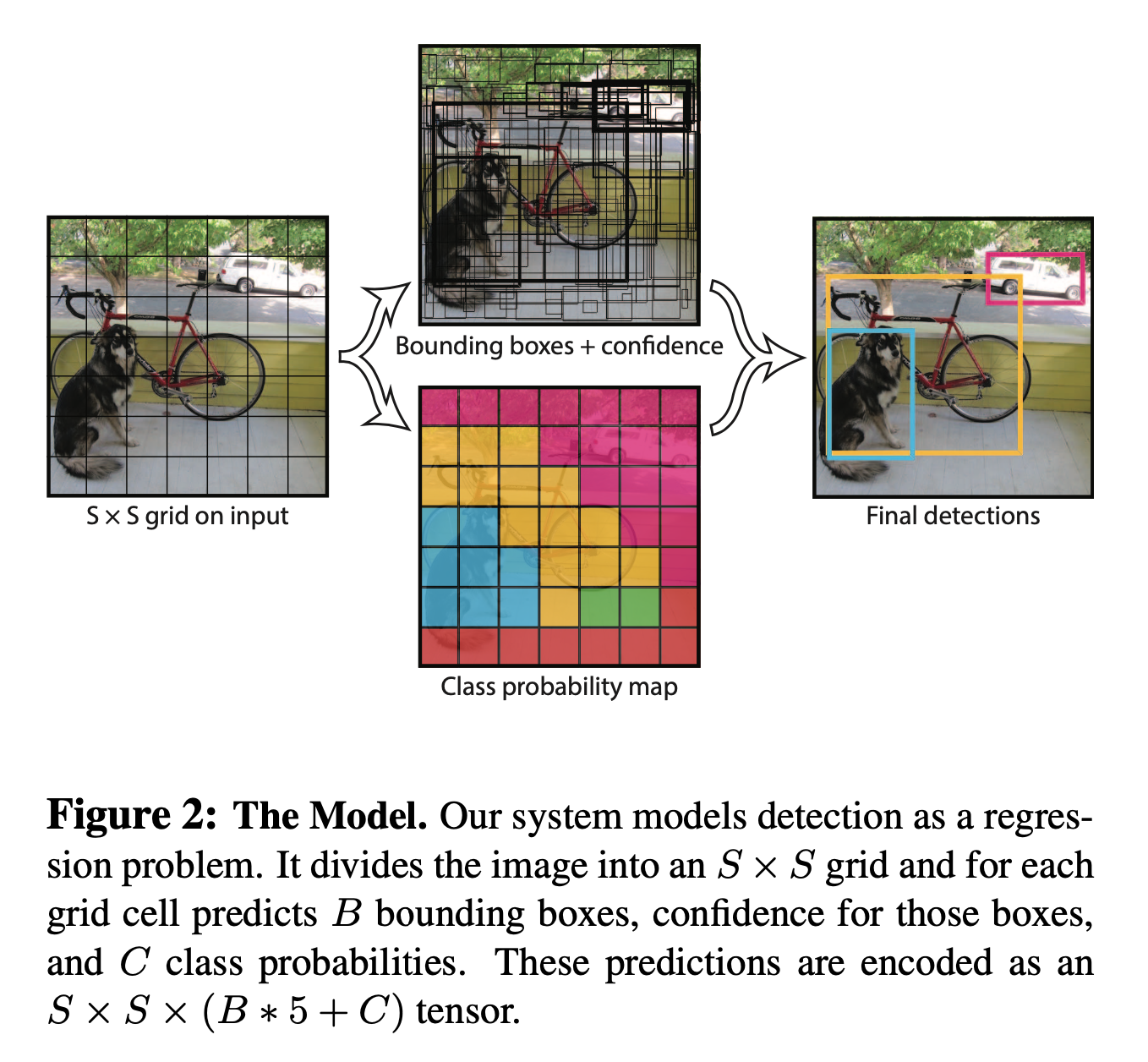6 YOLO Algorithm

# Unified Detection

The YOLO algorithm is primarily different in the unification of the detection and localization algorithm. Here, we’ll look at the algorithm itself.

S x S grid The input image is divided into an S x S grid. If the center of the object falls within that grid box, it is the boxes responsibility to claim the bounding box for that object. With an S x S grid, you can predict multiple bounding boxes on the same image.

B bounding boxes Each grid cell predicts B bounding boxes and confidence scores. The confidence score is the PR(Object) * IOU truth/pred. The IOU method is interesting becuase you only want to predict with confidence, that which your grid is able to see. Thus if you are able to see the center, in general you should be able to predict the class most confidently.

Predictions Each bounding box consists of 5 predictions x,y,w,h and confidence. (x,y) is the center, and w,h are relative to the whole image. The confidence is as shown before, a IOU between the predicted box and any ground truth box.

C Conditional Classes Each grid cell also predicts C conditional class probabilities. They are conditioned on the grid cell containing an object.

Figure 2 shows this process: